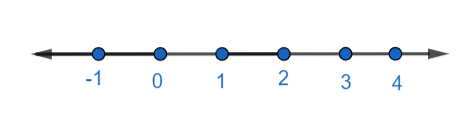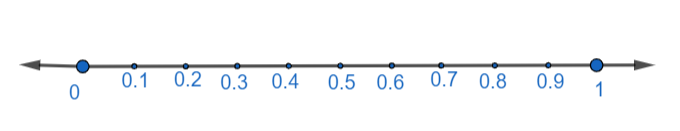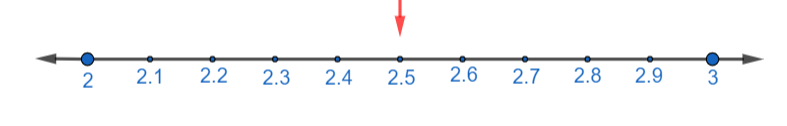QUESTION

# Show the following number on the number line.2.5

Hint: We will first see the concept of number line. Then we will check between which two integer numbers this point lies and then we will divide the number line between those two points to plot the given number.

Firstly, let us see what the number line is.

Number line is just a straight line on which points are marked at an equal interval of length, which represent numbers. In a number line, we can mark infinite numbers of points.
For example, we can plot number -1, 2, 3, 4 on number line as shown below:Also, lines between any two consecutive numbers can be divided into ten equal intervals. Where, each interval will be $\dfrac{1}{10}=0.1$ part of the length of interval of that two consecutive numbers. For example, we can divide number line between 0 and 1 further into ten intervals as:Of which, each interval can be further divided into ten equal intervals. This process can be repeated an infinite number of times, hence giving us infinite numbers between every two consecutive numbers.
Now, to plot 2.5 on the number line, we first check between which two consecutive numbers will lie 2.5.
So, we have,
$2<2.5<3$
That is, 2.5 lies between 2 and 3.

So, we will divide number line between 2 and 3 in ten equal intervals to plot 2.5 as below,Hence, 2.5 is plotted on a number line.

Note: If you know how to plot a fraction on a number line, then you can also choose to first convert the decimal number 2.5 into a fraction, which will be $2.5=\dfrac{25}{10}=\dfrac{5}{2}$ and then plot it on a number line.